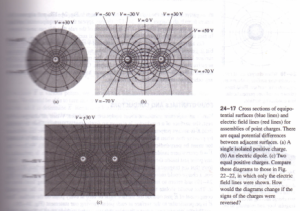# EQUIPOTENTIAL SURFACES

EQUIPOTENTIAL SURFACES

At various points in an electric field can be represented faces. These use the same fundamental idea as hikers and mountain climbers (Fig. 24-16). drawn through points that are all at the same drawn, but typically only a few contour lines are If a mass m is moved over the terrain along such a energy mgy does not change because the on a topographic map are really curves of co Contour lines are close together in regions where the changes in elevation over a small horizontal distance where the terrain is gently sloping. A ball allowed greatest downhill gravitational force where contour By analogy to contour lines on a three dimensional surface on which the electric pa test charge % is moved from point to point on energy % constant. In a region where an struct an surface through any point.Often with equal potential differences between be at two different potentials, so equipotential surfaces for never touch or intersect. “I energy does not change as a test charge moves over an field can do no work on such a charge. It follows that E must be ace at every point so that the electric force q) will always be . placement of a charge moving on the surface. Field lines and always mutually perpendicular. In general, field lines are curved surfaces. For the special case of a uniform field, in straight, parallel, and equally spaced, the equipotentials are to the field lines several arrangements of charges. The field lines in the plane of by red lines, and the intersections of the equipotential sure that is, cross sections of these surfaces) are shown as blue lines are three-dimensional. At each crossing of , the two are perpendicular have drawn so that there are equal potential differt surfaces. In regions where the magnitude of E is large, the are close together because the field does a relatively large amount in a relatively small displacement. This is the case near the point a or between the two point charges in Fig. 24-17b note that in lines are also closer together. This is directly analogous to the .ty being greatest in regions on a topographic map where Conversely, in regions where the field is weaker, the this happens at larger radii in Fig. 24-17a, to the left ofthe negative charge or the right of the positive charge in Fig. 24-1 stances from both charges in Fig. 24-17c. (It may appear that two.  intersect at the center of Fig. 24-17c, in violation of the rule that d.::l~a:::!:~'” In fact this is a single figure-8-shaped equipotential surface.)  n a given equipotential surface the potential V has the same but in general the electric-field magnitude E does not. For instance. surface labeled “V = -30 V” in Fig. 24-17b, the magnitude E negative charge than it is between the two charges. On the figure surface in Fig. 24-17c, E = 0 at the middle point halfway between any other point on this surface, E is nonzero.EQUIPOTENTIALS AND CONDUCTORS

Here’s an important statement about equipotential surfaces rest, the surface or a conductor is always an field E is always perpendicular to an equipotential surface, we  by proving that when all charges are at rest, the electric field r tor must be perpendicular to the surface at every point (Fig. E – 0 everywhere inside the conductor; otherwise, charges c any point just inside the surface the of E tangent to lows that the tangential component of E is also zero just not, a charge could move around a rectangular path partly inside 24-19) and return to its starting point with a net amount of it. This would violate the conservative nature of electrostatic component of E just outside the surface must be zero at every E is perpendicular to the surface at each point, proving our Finally, we can now prove a theorem that we quoted The theorem is as follows: In an electrostatic situation, if and if no charge is present inside the cavity, then there can be on the surface of the cavity. This means that if you’re inside you can safely touch any point on the inside walls of the box prove this theorem, we first prove that every point in the cavil) In Fig. 24-20 the conducting surface A of the cavity is an  have just proved. Suppose point P in the cavity is at a diff construct a different equipotential surface B including point P. Now consider a Gaussian surface, shown as a blue line, surfaces. Because of the relation between E and the field at every point between the equipotentials is from A toward! B” it is from B toward A, depending on which equipotential surface – either case the flux through this Gaussian surface is certainly  law says that the charge enclosed by the Gaussian surface diets our initial assumption that there is no charge in the cannot be different from that at the cavity wall. The entire region of the cavity must therefore be at the to be true, the electric field inside the cavity must be zero ~’0’1 law shows that the electric field at any point on the surface of a to the surface charge density (J at that point. We conclude on the wall of the cavity is zero at every point. This chain tortuous, but it is worth careful study.

CAUTION Don’t confuse equipotential surfaces with encountered in Chapter 23. Gaussian surfaces have relevance Gauss’s law, and we can choose any Gaussian surface that’s of equipotential surfaces; the shape is determined by the charge.